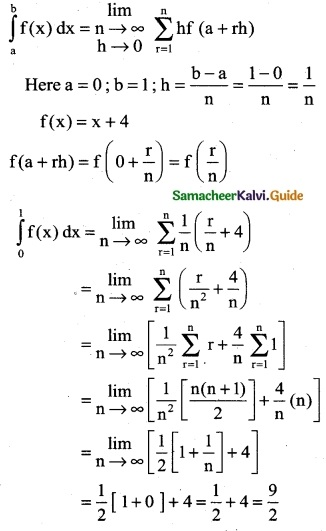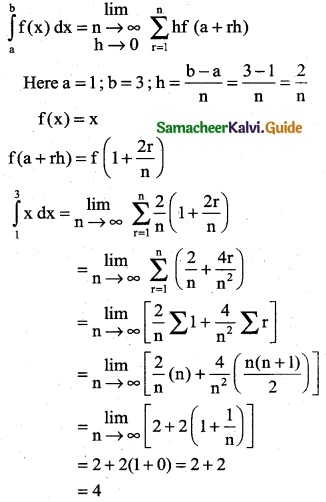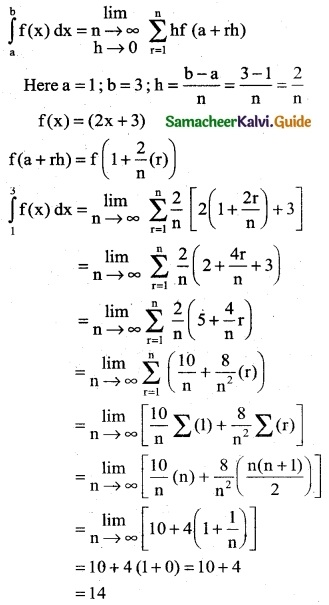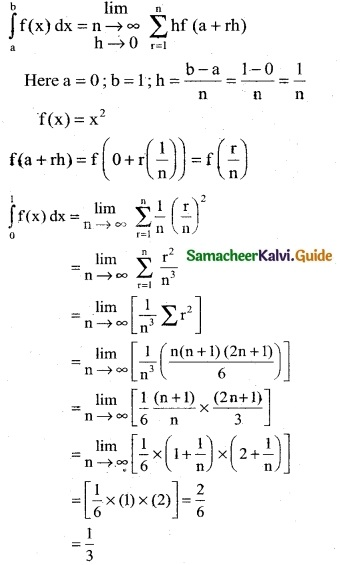Tamilnadu State Board New Syllabus Samacheer Kalvi 12th Business Maths Guide Pdf Chapter 2 Integral Calculus I Ex 2.11 Text Book Back Questions and Answers, Notes.

## Tamilnadu Samacheer Kalvi 12th Business Maths Solutions Chapter 2 Integral Calculus I Ex 2.11

Evaluate the following integrals as the limit of the sum:

Question 1.
$$\int_{0}^{1}$$ (x + 4) dx
Solution:Question 2.
$$\int_{1}^{3}$$ x dx
Solution:Question 3.
$$\int_{1}^{3}$$ (2x + 3) dx
Solution:Question 4.
$$\int_{0}^{1}$$ x² dx
Solution: